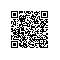# 给妹子讲python-S01E17函数的基本特征

【妹子说】那这一节我们先学习函数的最基本用法吧

def本身是一个可执行的语句，函数此时并不存在，直到python运行了def后，函数才存在，def后面包含的就是函数语句。在典型的操作中，def语句在模块文件中编写，并自然而然的在模块文件第一次被导入的时候生成定义的函数。

【妹子说】哎呀，说了这么些理论，也够枯燥的，那我们来看实际的例子吧：

def func(a,b):
return a+b

other_name = func
print(other_name(1,2))

3

python的函数使用还是非常简单的，我们再举一个例子：主要描述了两个方面：def定义（即函数的创建）和函数的调用（表达式告诉python去运行函数主体）

def func(x,y):
return x * y

print(func(2,4))

8

【妹子说】这和上面的例子不是一模一样吗？

def func(x,y):
return x * y

print(func(3.14,8))

25.12

-

def func(x,y):
return x * y

print(func('Ab',8))

AbAbAbAbAbAbAbAb

def func(x,y):
return x * y

print(func('Ab','aa'))

Traceback (most recent call last):
File "E:/12homework/12homework.py", line 4, in <module>
print(func('Ab','aa'))
File "E:/12homework/12homework.py", line 2, in func
return x * y
TypeError: can't multiply sequence by non-int of type 'str'

def func(seq1, seq2):
res = []
for x in seq1:
if x in seq2:
res.append(x)
return res

print(func([1,2,3,4,5,6], (5,6,7,8)))

[5, 6]

print(func('spam', ('s','p','c','m')))

['s', 'p', 'm']

print(func([1,2,3,4], [x for x in (2,3,5)]))

[2, 3]Python爱好者使用钉钉扫一扫加入圈子
+ 订阅